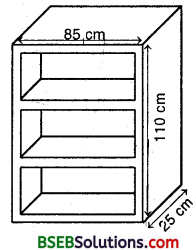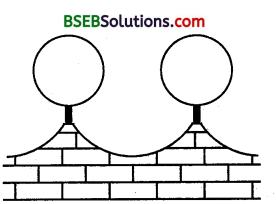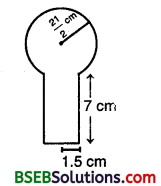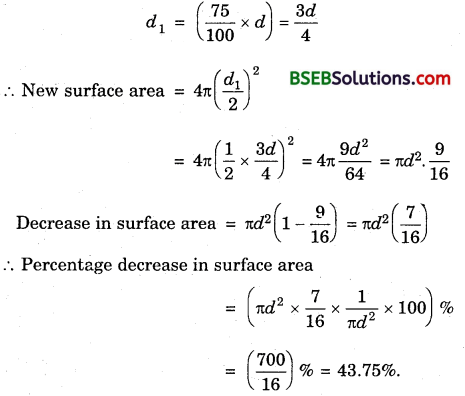# Bihar Board Class 9th Maths Solutions Chapter 13 Surface Areas and Volumes Ex 13.9

Bihar Board Class 9th Maths Solutions Chapter 13 Surface Areas and Volumes Ex 13.9 Textbook Questions and Answers.

## BSEB Bihar Board Class 9th Maths Solutions Chapter 13 Surface Areas and Volumes Ex 13.9Question 1.
A wooden bookshelf has external dimensions as follows : Height = 110 cm, Depth = 25 cm, Breadth 85 cm (see figure). The thickness of the plank is 5 cm everywhere. The external faces are to be polished and the inner faces are to be painted. If the rate of polishing is 20 paise per cm² and the rate of painting is 10 paise per cm², find the total expenses required for polishing and painting the surface of the bookshelf.Solution:
Area to be polished
= (110 x 85 + 2 x 85 x 25 + 2 x 25 x 110 + 4 x 75 x 5 + 2 x 110 x 5) cm²
= (9350 + 4250 + 5500 + 1500 + 1100) cm²
= 21700 cm²
Cost of polishing @ 20 paise per cm²
= (21700 x $$\frac { 20 }{ 100 }$$)
= Rs 4340
Area to be painted
= (6 x 75 x 20 + 2 x 90 x 20 + 75 x 90) cm²
= (9000 + 3600 + 6750) cm² = 19350 cm²
Cost of painting @ 10 paise per cm²
= Rs [ 19350 x $$\frac { 10 }{ 100 }$$] = Rs 1935
∴ Total expenses = Rs (4340 + 1935) = Rs 6275Question 2.
The front compound wall of a house is decorated by wooden spheres of diameter 21 cm, placed on small supports as shown in figure. Eight such spheres are used for this purpose, and are to be painted silver. Each support is a cylinder of radius 1.5 cm and . height 7 cm and is to be painted black. Find the cost of paint required if silver paint costs 25 paise per cm² and black paint costs 5 paise per cm².Solution:
Clearly, we have to subtract the part of the sphere that is resting on the sphere while calculating the cost of silver paint.
Surface area to be silver paint
= 8 (Curved surface area of the sphere – area of circle on which sphere is resting)
= 8(4πR² – πr²) cm², where R = $$\frac { 21 }{ 2 }$$ cm, r = 1.5 cm
= 8π(4 x $$\frac { 441 }{ 4 }$$ – 2.25) cm²
= 8π(441 – 2.25) cm²
= 8π(438.75) cm²
∴ Cost of silver paint @ 25 paise per cm²= Rs (8 x $$\frac { 22 }{ 7 }$$ x 438.75 x $$\frac { 25 }{ 100 }$$]
= Rs($$\frac { 19305 }{ 7 }$$) = Rs 2757.86 (approx.)
Surface area to be black painted
= 8 x curved surface area of cylinder
= 8 x 2πrh = 8 x 2 x $$\frac { 22 }{ 7 }$$ x 1.5 x 7 cm²
= 528 cm²
Cost of black paint @ 5 paise per cm² = Rs (528 x $$\frac { 5 }{ 100 }$$) = RS 26.40
Total cost of painting = Rs (2757.86 + 26.40) = Rs 2784.26 (appiox)Question 3.
The diameter of a sphere is decreased by 25%. By what percent does its curved surface area decrease?
Solution:
Let d be the diameter of the sphere.
Then, its surface area = 4π($$\frac { d }{ 2 }$$)² = πd²
On decreasing its diameter by 25%, the new diameter,